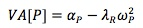### Why should I choose AnalystNotes?

Simply put: AnalystNotes offers the best value and the best product available to help you pass your exams.

### Subject 3. The active manager's objective

Objective: maximize the value added from residual return, where value added is measured as:where λR = aversion to residual risk. It transforms residual variance into a loss in alpha.

The loss in alpha increases as:

• The residual risk (i.e. ω2P) increases.
• The level of residual risk aversion (i.e. λR) increases.

The value added is also referred to as the certainty equivalent return.

#### Practice Question 1

The loss in alpha increases if ______ increases.

I. information ratio.
II. residual risk.
III. level of residual risk aversion.

#### Practice Question 2

The active manager's objective is to maximize the:

A. residual return.
B. information ratio.

#### Practice Question 3

An λ of 0.15 usually means ______ level of residual risk aversion.

A. low.
B. moderate.
C. high.